Games
Problems
Go Pro!

# Word Problems

Lesson Plans > Mathematics > Algebra > Word Problems

# Featured Lesson Plans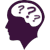Problems involving mixtures, including solutions, coins, populations - Algebra One Problems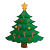A set of problems for students to solve - each related to ChristmasWord problems involving ages of people. Worksheet includes problem with both one, two or three people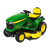Units cancellation technique for solving word problems - math problems in which two or more people work together to accomplish a task.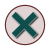Simple word problems, involving a single equation, and always using the word 'is' as the indicator for the equalsConverting a statement in a word problem into an equation can sometimes be simplified by rewriting the sentenceRecognizing the balance point of a sentence when the verb 'to be' shows up multiple timesSolving Algebra Word Problems - steps and examples for solving word problems in Algebra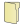# Full Directory Listing

Age Word Problems, Christmas Problem Solving, Mixture Problems, Rewriting Word Problem Statements, Steps to Solve Algebra Word Problems, Word Problem Worksheets 1, Word Problem Worksheets 2, Word Problem Worksheets 3, Word Problem Worksheets 4 (Geometry Formulas)Like us on Facebook to get updates about new resources